# Math Brain Teasers 8:: Lets Get Groovy - Find The Missing Number?(Edited)

Hey All;

I hope you all are liking the Math Brain Teasers. If there is any feedback you have, I'm open to having them discussed and make changes accordingly to the competition that we are having here on the StemGeeks community daily.

I guess in between some of the Math puzzles got a bit complicated. So this time we have an easy and interesting puzzle to solve. Have a look at the following image and try finding out the missing number that should be replaced by the question mark.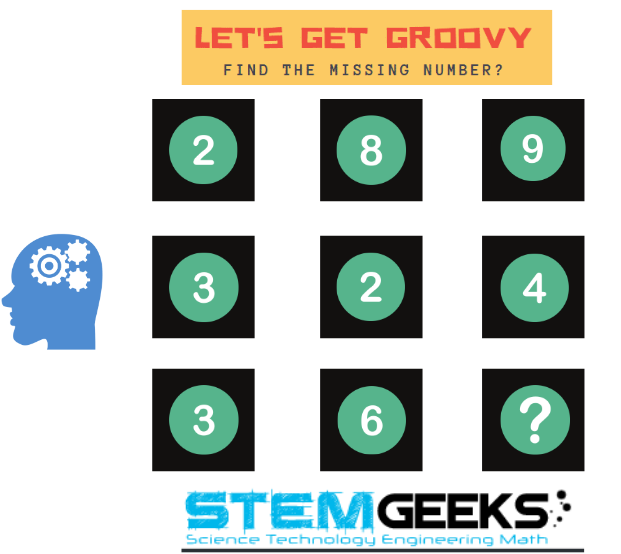Hint:: I think that I shouldn't be giving any Hint for this Brain teaser as it's a simple one. And giving Hint here will make it super easy, which I don't want it to be.

##### Math Brain Teasers 7:: Solve The Triangle Puzzle - Solved with Explanation

Let's have a look at the answers and the math/logic involved in finding to:: Solve The Triangle Puzzle? for our SEVENTH Math Brain Teasers 7 Question.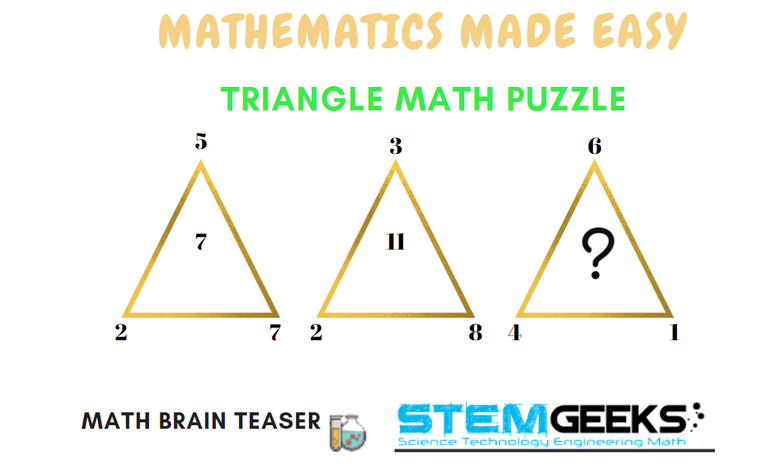### Logic to Solving:: The Triangle Puzzle?

Following is the logic used in solving THe Triangle Math Puzzle. You will need to use the equation::

#### D = 2xC-(A+B)

as your base to get to find the missing number in the Third Triangle.

where D is the central number that we are looking to find. And **A, B, C ** are the numbers around the triangle in an anti-clockwise direction starting from the top of the triangle. This was the HINT that I had already given with regards to anti-clockwise direction.

#### Steps to decode Tringle 1 Answer

A = 5 B = 2 C= 7

Equation:: D = 2xC-(A+B)

D = 2*7 - (5+2)

D = 14 - 7 = 7

#### Steps to decode Tringle 2 Answer

A = 3 B = 2 C= 8

Equation:: D = 2xC-(A+B)

D = 2*8 - (3+2)

D = 16 - (5)

D = 11

#### Steps to decode Tringle 3 Answer

A = 6 B = 4 C= 1

Equation:: D = 2xC-(A+B)

D = 2 * 1 - (6 + 4)

D = 2 - 10

D = -8

Hence the correct answer to our **Math Brain Teasers 7 :: Solve The Triangle Puzzle = -8

### STEM token GiveAway

I'll be again doing a giveaway of STEM tokens to the lucky random winner with the correct answer. We had 9 entries & out of which 1 entry was correct and that is of @pimpstudio-cash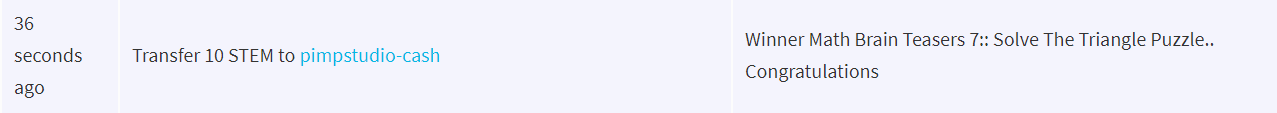Congratulations @pimpstudio-cash; Your WIN yourself 10 STEM tokens; worth 2.2+ HIVE. You should be having the rewards in your STEM Wallet Soon.

### Math Quote for the Day::

Here is the motivation to solve this Math Brain Teasers 8:: Find The Missing Number?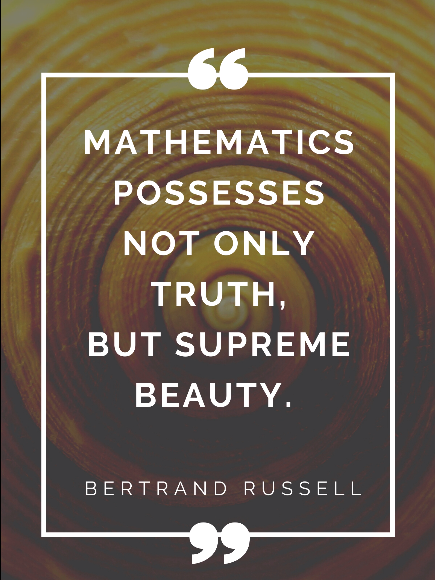If you like my work, then please spread the Word.. that we do have the Math Brain Teasers competition here @StemGeeks platform. Reblog is much appreciated.

Best Regards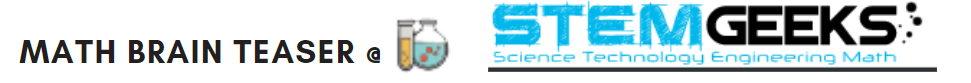PS:: All the Maths Brain Teasers; are made by me using the Pro Canva License Version

Posted with STEMGeeks

0
0
0.000I'm guessing 8?

0
0
0.0000
0
0.000My brain is telling it's 8 .

0
0
0.0000
0
0.0000
0
0.000(Edited)

For me in first try are 11 . i try other solution but for now that is :) thankx for the last math brain teaser
but here are posibile 2 solution and 8 are posibile . :(((( horizontally or vertically and both have logic

0
0
0.000My guess is 1 😀
Best of luck to everyone 🤞

0
0
0.000thanks @gungunkrishu for sharing another post with the WINE community!
As always we've upvoted it and toast to your post. Cheers!

TEAM !WINE

0
0
0.000Cheers, @wine-token You Successfully Shared 0.100 WINE With @gungunkrishu.
You Earned 0.100 WINE As Curation Reward.
You Utilized 1/3 Successful Calls.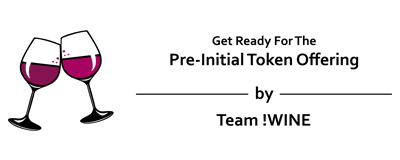WINE Current Market Price : 0.000 HIVE

0
0
0.000